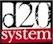SRD Homed20 Modern System Reference Document

## The Basics

### DICE NOTATION

These rules use the following die notations:
d4 = four sided die
d6 = six sided die
d8 = eight sided die
d10 = ten sided die
d12 = twelve sided die
d20 = twenty sided die
d% = percentile dice (a number between 1 and 100 is generated by rolling two different ten-sided dice. One (designated before rolling) is the tens digit. The other is the ones digit. Two 0s represent 100.)

Die rolls are expressed in the format:
[#] die type [+/- modifiers]
Example: 3d6+2 means: "Roll 3 six sided dice. Add the result of the three dice together. Add 2."

##### ROUNDING FRACTIONS

In general, if you wind up with a fraction, round down, even if the fraction is one-half or larger.
Exception: Certain rolls, such as damage and hit points, have a minimum of 1.

##### MULTIPLYING

Sometimes a special rule makes you multiply a number or a die roll. As long as you’re applying a single multiplier, multiply the number normally. When two or more multipliers apply, however, combine them into a single multiple, with each extra multiple adding 1 less than its value to the first multiple. Thus, a double (x2) and a double (x2) applied to the same number results in a triple (x3, because 2 + 1 = 3).